Y9 Progress Check 9.1
Variables, data types and binary
First Name: *
Last Name *
Class: *
What Python Programming Challenge are you currently on? *
What does IDE stand for?
1 point
In 1991 Guido van Rossum created the Python programming language. Why did he call it Python?
1 point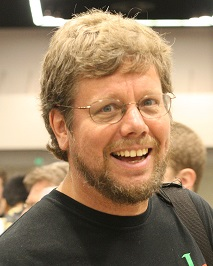Which description best describes a variable?
1 point
Which data type would be most appropriate if you were assigning the following information to a variable?
5 points
String
Integer
Float
Boolean
Name
Age (Years)
Postcode
Student (Y/N)
Height (Meters)
Select the lines of code that will not cause a syntax error?
1 point
If number = 2 and fruit = "apples", what would these print() functions output?
5 points
2 apples
Error
number, fruit
applesapples
print(number+apples)
print(number,fruit)
print(number*fruit)
print(number+fruit)
print("number, fruit")
What will this code output when it is run?
1 point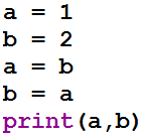With variables, readability is very important. Which of the following is easiest to read? They are all valid variable names.
1 point
What data type is my_variable when this code is run?
1 point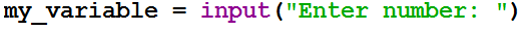Which line(s) of code will successfully calculate the area of a triangle of base (b) and height (h)?
1 point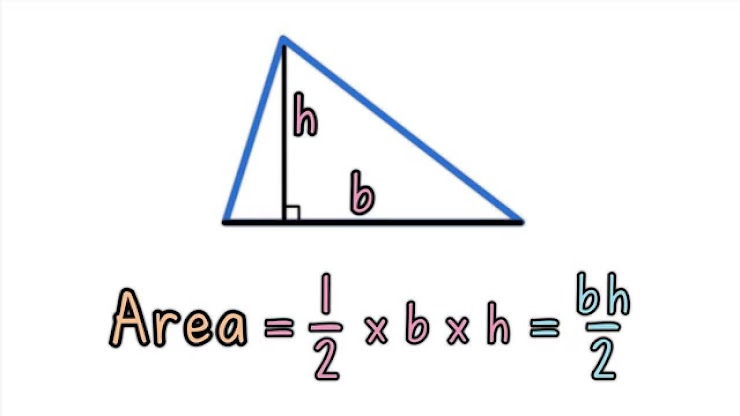In the question above, what data type will the variable 'area' be if the correct code is used?
1 point
What data type is my_variable when this code is run?
1 point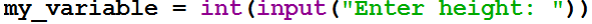What is the smallest unit of information that a computer can store?
1 point
What are 8 Bits called?
1 point
How many Bits in a Nibble?
1 point
What is 13 in 4 bit binary?
1 point
What is 99 in 8 bit binary?
1 point
Binary addition: What is 1010 + 0101 ?
1 point
Binary addition: What is 1100 + 1101 ?
1 point
Fill in the blank: Computers count in binary as they are made up of millions of _______ that can either be off or on, this is represented as a 0 or a 1.
1 point